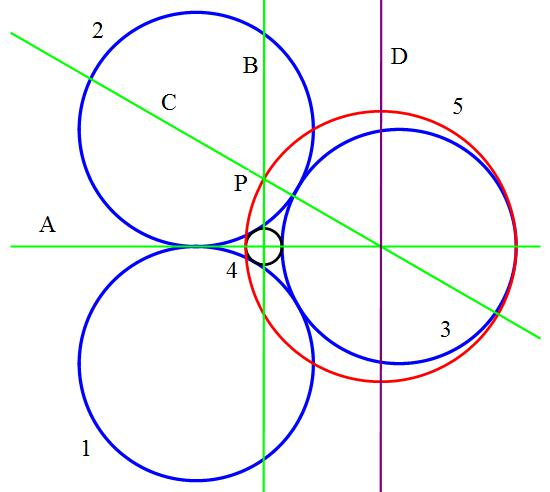# You can guess but can you prove it?

Geometry Level 2

Identical circles, $1,2,3$, tangent to each other, are tangent to inner circle $4$.Circle $5$ is tangent to circles $3,4$ inside it. Line $A$ passes through the centers of circles $3, 4, 5$. Line $B$, perpendicular to Line $A$, passes through the center of circle $4$, and intersects circle $5$ at point $P$. Line $C$ passes through point $P$ and the center of circle $5$.

Find the small angle between lines $A$ and $C$ in degrees.

Note: Line $C$ does not pass through the centers of circles $2$ and $3$!

×